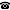# 2008 seminar talk: A remark on a theorem by Hajnal and Juhasz

Talk held by Bernhard Irrgang (Universität Bonn) at the KGRC seminar on 2008-03-13.

### Abstract

By a theorem of Hajnal and Juhasz, $card(X)\leq exp(exp(spread(X)))$ holds for all Hausdorff spaces $X$. Juhasz asked, if the second $exp$ is necessary. I will present a result which shows that $card(X)=exp(exp(spread(X)))$ for a $T_3$ space $X$ with spread $\omega_1$ is consistent. Previously, Fedorcuk constructed from $\diamondsuit$ a $T_3$ space $X$ with spread $\omega$ and $card(X)=exp(exp(spread(X)))$.

Kurt Gödel Research Center for Mathematical Logic. Währinger Straße 25, 1090 Wien, Austria.+43-1-4277-50501. Last updated: 2010-12-16, 04:37.